# Level Order Traversal of a Binary Tree :  Breadth First Search

### Introduction to level order traversal

In the DFS traversal of a binary tree, we access nodes in three different orders — preorder, postorder and inorder. Now we have another traversal that accesses nodes in level by level order. This is called level order traversal or breadth-first search traversal. In the short form, we also call it BFS traversal.

A binary tree is organized in different levels where the root node is at the topmost level (0th level). So we start from the root node and process it, then process all the nodes at the first level, then process all the nodes at the second level, and so on. In other words, we explore all nodes at the current level before moving on to the nodes at the next level.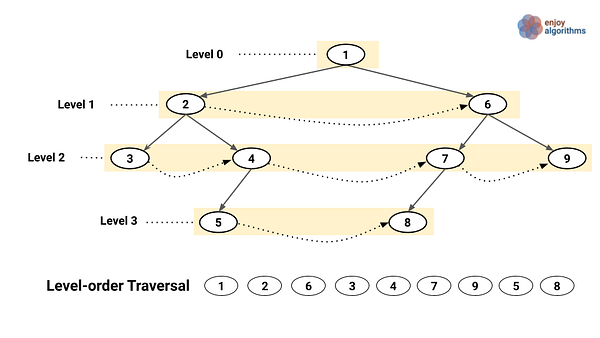### The recursive approach of BFS traversal

This is a brute force idea where we go through each level using a loop and process nodes at each level using recursion. The idea is simple, but implementation is a little tricky. The objective to discuss this approach is related to problem-solving. In the recursive solution of few tree problems, sometimes we pass extra parameters or use a helper function to generate the correct output. Let’s think about the implementation of the recursive BFS traversal:

• The number of levels is equal to the height of the tree. So we first calculate the height of the tree h using the function height(root).
• We run a loop from l = 0 to h  -  1 to access each level in the tree. Inside the loop, we use the function processCurrentLevel(root, l) to visit the current level l and process the nodes at that level.

How we implement the function processCurrentLevel(root, l)? Let’s think! We can split the problem into two parts:

• We process all the nodes at level l present in the left subtree. For this, we call the same function with root->left and l - 1 as a function parameter. Why? Because, if the level is l distance from the root, then it would be l-1 distance away from the root->left.
• We process all the nodes at level l present in the right subtree. For this, we call the same function with root->right and l - 1 as a function parameter. Why? Because, if the level is l distance from the root, then it would be l -1 distance away from the root->right.
• When l==0 during the recursive calls, we arrived at a node which is distance l from the root. We process this node. In such a way, we can access all the nodes at the level l recursively.

Pseudocode Implementation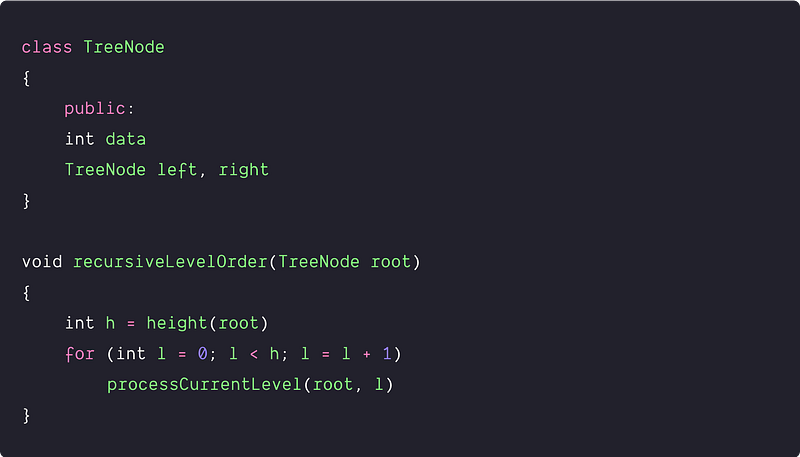Processing nodes at the level l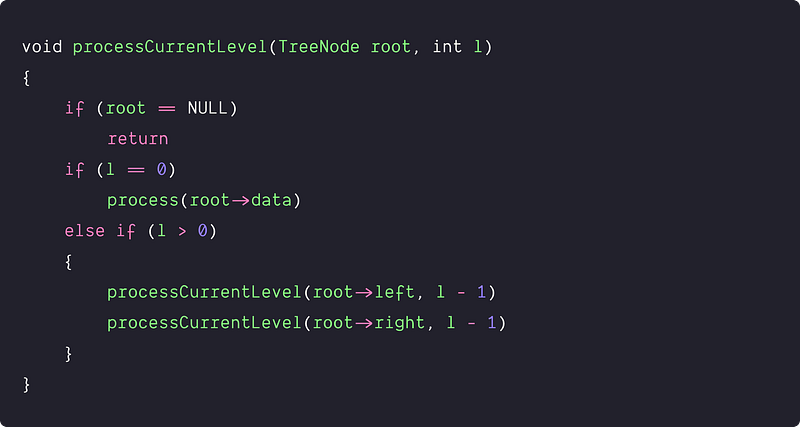Calculating the height of the tree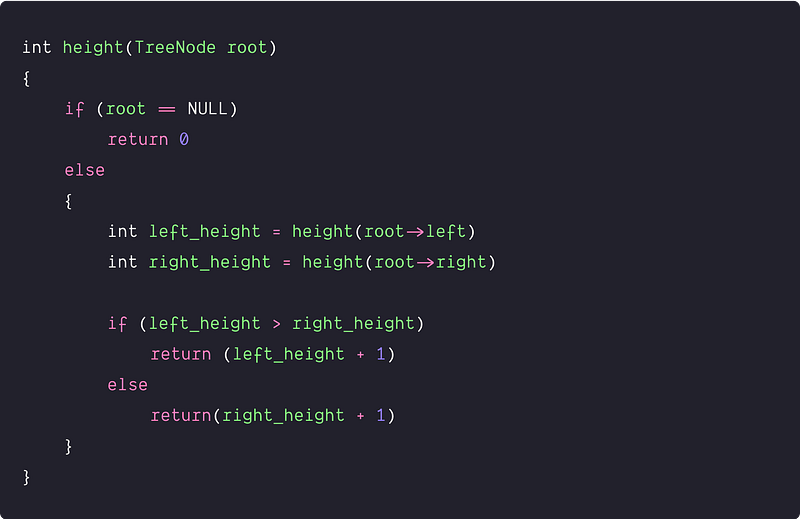Time and space complexity analysis

For each function call processCurrentLevel(), we are going l -1 level down in the left and right subtree. In other words, we are accessing each node from level 0 to level l and checking if l==0 or not. So one idea is clear: the total number of operations depends on the height of the tree. Think!

Worst case scenario would be the skewed tree where one node is present at each level. In this case, processCurrentLevel() takes O(n) time for the last level, O(n-1) time for the second last level, and so on. Here n is the number of nodes in the skewed tree.

Time complexity = O(n) + O(n-1) + .. + O(1) = O(n + (n-1) + ... + 1) = O(n^2).

Critical question: What would be the best case analysis? Think! Space complexity: O(n) in the worst case. For a skewed tree, processCurrentLevel() uses O(n) space for the call stack. For a balanced tree, the call stack uses O(log n) space, (i.e., the height of the balanced tree).

### An efficient approach: BFS traversal using queue

Now the critical question is — can we optimize the time complexity of the BFS traversal? Can we traverse tree level by level in O(n) time complexity? Think!

Let's observe the order of nodes in level order traversal. We first process the root node at level 0, and then we process the left and right child at level 1 (assuming the order from left to right). Similarly, at the second level, we first process the children of the left child of the root then process the children of the right child. This process goes on for all the levels in the tree. So one idea is clear: at any level, the node which will be processed first at any given level, the children of that node will be processed first at the next level. So this is the First In First Out order of processing nodes, and we can think to use the queue data structure to simulate the BFS traversal.

Implementation steps: BFS traversal using queue

1. We take an empty queue treeQueue and initialize it with the root node.
2. Now we run a loop till treeQueue is not empty. Inside the loop, we declare a currNode to track the current node during the traversal.
3. We start the loop by deleting the front node from the treeQueue and assign it with currNode. We process the currNode and insert the left and right child into the treeQueue.

``````TreeNode currNode = treeQueue.dequeue()
process (currNode->data)
``````
4. If the left child of currNode is not NULL, we insert the left child into the treeQueue.

``````if (currNode->left != NULL)
treeQueue.enqueue(currNode->left)
``````
5. If the right child of currNode is not NULL, we insert the right child into the treeQueue.

``````if (currNode->right != NULL)
treeQueue.enqueue(currNode->right)
``````

Pseudocode  of BFS traversal using queue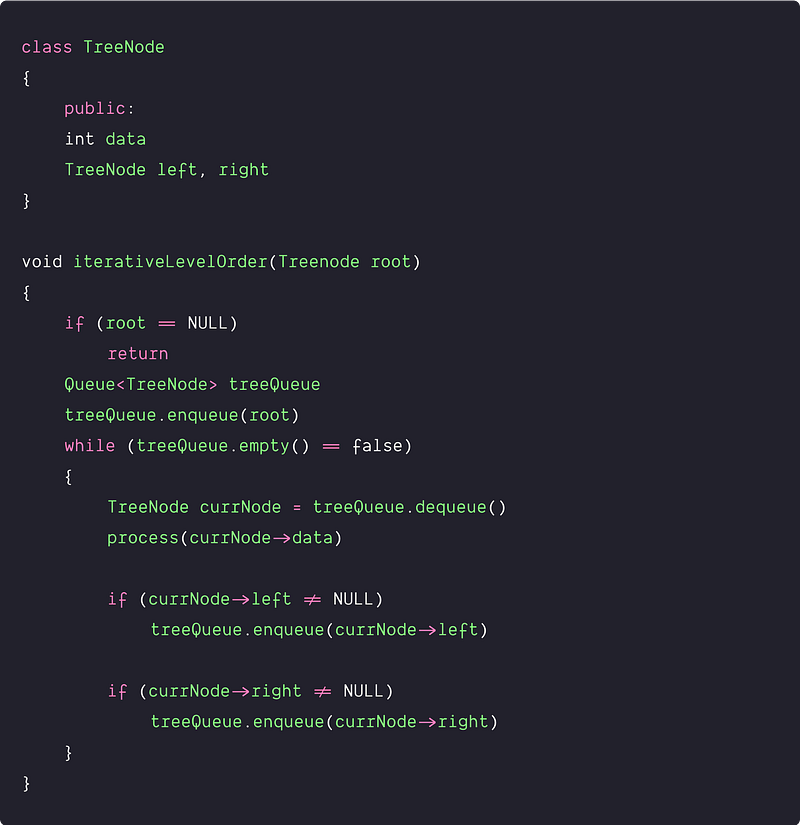Time complexity analysis of the BFS traversal

• Suppose n number of nodes are given in the input.
• The time complexity of enqueue and dequeue operations = O(1)
• We are doing two queue operations for each node inside the loop— inserting once into the queue and deleting once from the queue. So total count of queue operations = 2n.
• So overall time time complexity = total queue operations * time complexity of each queue operation = 2n * O(1) = O(n)

The space complexity analysis of the BFS traversal

Space complexity is equal to the queue size. We process nodes level by level, so max queue size depends on the level with a maximum number of nodes or max-width of the binary tree. If the maximum width of the binary tree is w, then space complexity = O(w). The idea is simple: the width of w depends on the structure of the given binary tree. How? Let’s think!

Worst case: When the tree is balanced

When the tree is balanced, the last level will have the maximum width or the maximum number of nodes, which will be 2^h (where h is the height of the tree). For a balanced tree, h = logn and required size of the queue = O(2^h) = O(2 ^ (log n)) = O(n). So space complexity = O(n)

Best case: When the tree is a skewed tree or a linked list

In such a case, every level will have a maximum of one node, and thus at any point of time, there will be at most one node in the queue. So required size of the queue = O(1). So space complexity = O(1)

### BFS vs DFS traversal of binary tree

• Both traversals require O(n) time as they visit every node exactly once.
• Depth-first traversal starts from the root, goes to the depth as far as possible, and then backtracks from there. In other words, it visits nodes from the bottom of the tree. But in breadth-first search, we explore nodes level by level i.e. in the order of their distance from the root node. So if our problem is to search for something closer to the root, we would prefer BFS. And if we need to search for something in the depth of the tree or node closer to a leaf, we would prefer DFS.
• In BFS traversal, we use the queue data structure to stores nodes of different levels. But in DFS traversal, we use the stack (If recursive, system use function call stack) to stores all ancestors of a node.
• The memory taken by both BFS and DFS depends on the structure of the tree. Extra space required for BFS Traversal is O(w), but additional space needed for DFS Traversal is O(h). Here w = maximum width of binary tree and h = maximum height of the binary tree. In the worst case, both require O(n) extra space, but worst cases occur for different types of trees. Additional space needed for BFS Traversal is likely to be more when a tree is more balanced, and extra space for DFS Traversal is likely to be more when a tree is less balanced.
• Sometimes, when node order is not required in problem-solving, we can use both BFS and DFS traversals. But in some cases, such things are not possible. We need to identify the use case of traversal to solve the problems efficiently.

### Problems to practice using BFS traversal

• Level order traversal in spiral form
• Reverse Level Order Traversal
• Left View of Binary Tree
• Maximum width of a binary tree
• Min Depth of Binary Tree
• Level with the maximum number of nodes
• Count the number of nodes at a given level
• Convert a binary tree into a mirror tree

Enjoy learning, Enjoy coding, Enjoy algorithms!

Get well-designed application and interview centirc content on ds-algorithms, machine learning, system design and oops. Content will be delivered weekly.